Students get through the MP Board Class 11th Physics Important Questions Chapter 5 Laws of Motion which are most likely to be asked in the exam.

MP Board Class 11th Physics Important Questions Chapter 5 Laws of Motion

Laws of Motion Class 11 Important Questions Very Short Answer Type

Question 1.
What is inertia? What are its types?
The property of the bodies by virtue of which the bodies are unable to change their state of rest or motion, is called inertia.
Inertia is of two types :

1. Inertia of rest and
2. Inertia of motion.
• Inertia of rest: If a body is at rest it will remain at rest until and unless an external force is applied on it. This property of the body is called inertia of rest.
• Inertia of motion : If a body is in motion it will continue its motion until and unless an external force is applied. This property of the body is called inertia of motion.

Question 2.
How the inertia of a body depends upon the mass of a body?
Inertia of a body is directly proportional to its mass.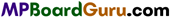Question 3.
What is force ? What are its effects ?
Force: The force is an external agent which produces or tends to produce change in the state of rest, motion, shape or size of a body.
Effect of force :

• Change in state of motion.
• Change in state of rest.
• Change in shape or size.

Question 4.
What will be the magnitude of the resultant force acting upon a body which is in uniform motion?
Force upon the body will be zero because, when velocity remains constant, the acceleration of the body is zero.

Question 5.
What do you mean by momentum?
The product of mass and velocity of a body is called its momentum.
Momentum = Mass × Velocity.

Question 6.
Write the laws of inertia.
Newton’s first law of motion : If a body is at rest, it will remain at rest and if it is in motion, it will continue its motion, until and unless an external force is acted upon it.

Question 7.
Define 1 newton of force.
The force required to produce an acceleration of 1 ms-2 on a body of mass 1 kg, is called 1 newton.

Question 8.
What is impulse?
When a greater force is applied for small interval of time on a body, then the product of force and time interval is called impulse.
Impulse shows the effect of force, i. e.,
I = Ft.

Question 9.
A cricket player moves his hand backward while he catches the ball. Why?
Impulse of a force is given by FΔt = ΔI
∴ F = $$\frac{\Delta I}{\Delta t}$$
As Δt increases, force F decreases. Therefore, while taking the catch, the time interval Δt increases when he withdraws his hands, thus F decreases and player is not injured.Question 10.
How do the man walk on earth?
The man presses the earth in the backward direction, as a result, by Newton’s third law, the earth applies an equal and opposite force in the forward direction. It is due to reaction force, the person moves in the forward direction.

Question 11.
When a gun is fired it gives backwards jerk. Why?
When the bullet is fired from the gun, the bullet moves with high velocity and due to its reaction, a force acts on the gun in opposite direction and hence, it gives jerk.

Question 12.
Jumping on the hard floor is hurtful but not on the sand. Why?
When a man jumps on a hard floor, the legs come to rest immediately, therefore value of Δt is very less, which increase the force and hence, the jumping becomes hurtful than on the sand.

Question 13.
Shock absorber are used in vehicles. Why?
Shock absorber increases At. At the time of jerks, the force F decreases. Thus, the driver of vehicle receives less jerks.

Question 14.
What is Newton’s third law of motion?
According to Newton’s third law of motion, “Every action has equal and opposite reaction.”
$$\vec{F}_{21}=-\vec{F}_{12}$$

Question 15.
Action and reaction are equal and opposite, do they cancel each other. Why?
Action and reaction do not cancel each other because both act on different bodies.

Question 16.
What are concurrent forces? What is the condition of their equilibrium ?
When the lines of action of two or more forces pass through a point, then the forces are called concurrent forces.
Let $$\vec{F}_{1}, \vec{F}_{2}, \vec{F}_{3}, \ldots, \vec{F}_{n}$$ forces are acting on a point, then the condition of equilibrium is
$$\vec{F}_{1}+\vec{F}_{2}+\vec{F}_{3}+\ldots+\vec{F}_{n}=0$$

Question 17.
What is absolute unit of force?
1 Newton = 1 kg × 1 m/s2
and 1 Dyne = 1 g × 1 cm/s2.

Question 18.
Which is the fundamental laws of motion and why?
Newton’s second law is the fundamental laws of motion because first law and third laws can be derived from it.Question 19.
What has been specified by Newton first law of motion?
Newton first law defines force and inertia.

Question 20.
A passenger sitting in a bus at rest pushes it from within will it move. Why?
No, internal forces are unable to produce motion in a system.

Question 21.
According to Newton’s third laws of motion, every force is accompanied by an equal and opposite force called reaction, then how can a movement take place?
As the action and reaction never act on the same body, so the motion is possible.

Question 22.
A stone when thrown on a glass window smashes the window pane to pieces. But a bullet fired from a gun passes through it making a hole, why?
This is due to inertia at rest.

Question 23.
Explain why jet planes cannot move in air free space but rockets can move?
Jet plane uses atmospheric oxygen for their fuel but rockets carry their own fuel and don’t depend on atmospheric oxygen.

Question 24.
What are the absolute unit of force ? Write its definition?
Newton is the absolute unit of force in S.I. unit.
1 Newton = 1 kg × 1 m/s2
The force requird to produce an acceleration of 1 m/s2 in a body of mass 1 kg is called one Newton.
In C.G.S unit the absolute unit is dyne, 1 dyne = 1 g × 1 cm/s2
It is defined as the force required to produce an acceleration of 1 cm/s2 in a body of mass 1 gram
1 Newton = 1 kg × 1 m/s2
= 1000 g × 100 cm/s2
= 105 g-cm/s2
or 1 Newton = 105 dyne
This is the relation between Newton and dyne.

Question 25.
A body is moving with uniform velocity. Does force is neccessary to maintain constant velocity?
No, according to the first law of motion velocity reamains constant in the presence of force.

Question 26.
A constant force is acting upon a body. Among velocity, acceleration and kinetic energy, which quantity remain conserved and which will change ?
Acceleration will remain conserved.
Since acceleration = $$\frac{\text { Constant force }}{\text { Constant mass }}$$
Velocity and kinetic energy will get changed.Question 27.
What is friction force ? What is its nature?
When there is a relative motion between two surfaces in contact, then an opposing forces acts between the surfaces, is called friction force.
Its nature is that it always opposes the motion.

Question 28.
Ball bearings are used to reduce friction. Why?
The rolling friction is lesser than that of sliding friction, therefore ball bearing are used to reduce friction.

Question 29.
How many types of friction are there ?
Friction are of two types :

1. Static friction,
2. Kinetic friction.

Question 30.

• We can walk on ground due to friction.
• We can stop vehicle by applying brakes due to friction.
• We get fire due to friction.
• We can chew food due to friction.

Question 31.

• Machinary parts get damaged due to friction.
• Efficiency of machine decreases.
• Some part of energy given to machine get lost in form of heat.

Question 32.
How can we reduce friction?

• By polishing,
• Ball bearing,
• By greasing.

Question 33.
A body of mass m is rotated in the circular paths of radii r1 and r2 with the velocities ν1 and ν2. If r1, > r2, then which will be greater ν1 or ν2, supposing that same centripetal force is required for the motion.
As the centripetal forces are equal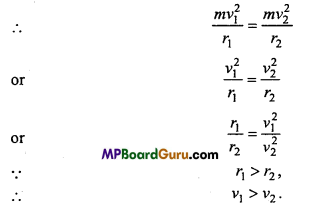Question 34.
A body is moving in a circular path with uniform speed. If its frequency is n and radius of path is r, then write the formula of centripetal acceleration in terms of r and n.
Centripetal acceleration = rω2 = r(2πn)2
= 4π2n2r.Question 35.
Define centripetal force.
When a body moves in a circular path, a force acts towards the centre of the path, which revolves the body, is called centripetal force.
Centripetal foree = mνω=mω2r = $$\frac{m v^{2}}{r}$$

Question 36.
Derive the dimension formula of centripetal force by its formula.
Centnpetal force = $$\frac{m v^{2}}{r}$$
=$$\frac{[\mathrm{M}]\left[\mathrm{LT}^{-1}\right]^{2}}{[\mathrm{~L}]}$$
= $$\frac{[M]\left[L^{2} T^{-2}\right]}{[L]}=$$
= [MLT-2].

Question 37.
If the velocity and radius of circular path, both are doubled, then what will be the change in centripetal force?
Centripetal force = $$\frac{m v^{2}}{r}$$
When the radius and velocity are doubled, then
centripetal force = $$\frac{m(2 v)^{2}}{2 r}=\frac{4 m v^{2}}{2 r}=\frac{2 m v^{2}}{r}$$
Hence, the centripetal force will be doubled.

Question 38.
Milk is stirred, to separate the cream. Why?
The cream particles are lighter than milk particles. Therefore, cream particles need lesser centripetal force to move in circular path. When the milk is stirred both type of the particles receive same centripetal force, hence the cream particles move in the circular paths of lesser radii than that of milk particles. Thus cream particles are separated.

Question 39.
Why the roads are banked at the turnings?
Or
The outer rails are raised at the turnings. Why?
The reasons are :

• It prevents the vehicle from skidding.
• The speed of vehicle should be greater at the turnings.
• Least damage to the tyres by friction.

Question 40.
A particle is moving with uniform speed in a circular path. What is the nature of its acceleration?
The acceleration acts towards the centre, perpendicular to the direction of velocity.

Question 41.
The circular roads on the hills are banked out sider. Why?
The roads are banked outside to prevent the vehicle from skidding, the speed can be more and the tyres are less damaged by friction.

Question 42.
The wings of an aeroplane lean inwards while taking turn. Why?
The wings of an aeroplane lean inwards to provide necessary centripetal force to moves in a circular path.Question 43.
It is easy to rotate a stone with smaller string than longer string. Why?
The necessary centripetal force to rotate in a circular path is provided by the tension of the string. For smaller string, less centripetal force is required to rotate, which can be provided easily by applying less force.

Question 44.
A man sitting in a moving bus dashes against the window, when the bus takes turn. How does the man explain this accident and how another man standing outside the bus will explain?
A man sitting in the bus will think that the reaction force of centripetal force, acted on him. While the person standing outside will think that the man dashes against the window due to inertia of motion.

Laws of Motion Class 11 Important Questions Short Answer Type

Question 1.
State Newton’s laws of motion.
First Law : A body can’t change its state of rest or of uniform motion along a straight line unless an external force acts on it.
Second Law : The rate of change of momentum is directly proportional to the impressed force and directed towards the direction of force.
Third Law : For every action there is equal and opposite reaction.
$$\vec{F}_{21}=-\vec{F}_{12}$$

Question 2.
What is law of conservation of momentum? Prove it.
Law of conservation of momentum: In the absence of external force the linear momentum of a system remains always constant.
Proof: Let a force $$\vec{F}$$ is applied on a system for time interval dt, so that its momentum changes by dp.
∴ By Newton’s second law, F = $$\underline{d p}$$
If the system is in equilibrium, then the sum of all the forces acting on it will be zero,
F = 0
∴ $$\frac{d p}{d t}$$= 0
or p = constant, (by differential calculus, that $$\frac{d}{d t}$$ const. = 0)
Thus, in the absence of external force the linear momentum of a system is always conserved.

Question 3.
Define momentum. If two bodies are moving with equal velocities, then the momentum of the heavier body will be more than that of lighter body.
Momentum is the product of mass and velocity of a body :
(i) If the masses of a heavier body and a lighter body are M and m respectively and if they are moving with equal velocities ν, then
Momentum of the heavier body
P1 = Mν … (1)
and the momentum of the lighter body
p2 = mν … (2)
On dividing the eqn. (1) by eqn. (2), we have
$$\frac{p_{1}}{p_{2}}=\frac{M v}{m v}$$
or $$\frac{p_{1}}{p_{2}}=\frac{M}{m}$$ ….(3)
But M > m
∴ P1 > p2
Clearly, if two bodies are moving with equal velocities, then the momentum of the heavier body will be more than that of the lighter body.

Question 4.
If two bodies are having same momentum, then velocity of lighter body will be more than velocity of heavier body.
Let the mass of heavier body be M and lighter body be m. Momentian of both bodies be p. Let’s their velocities be ν1 and ν2
Then, Momentum of heavier body = Mν1
and Momentum of lighter body = mν2
Since Momentum of both bodies are same.
1 = mν2
or
$$\frac{v_{2}}{v_{1}}=\frac{M}{m}$$
Since M > m ∴ ν2 > ν1 i.e., velocity of lighter body is more than that of velocity of heavier body.

Question 5.
Write Newton’s second law of motion and prove that force = mass x acceleration.
Newton’s second law of motion : The rate of change of momentum is directly proportional to the force applied and directed towards the direction of force.
Let the initial velocity of a body of mass m is u.
∴ Initial momentum $$\overrightarrow{p_{1}}=m \vec{u}$$
If force $$\vec{F}$$ is applied for time Δt, so that its velocity becomes $$\vec{v}$$.

∴ Final momentum, $$\vec{p}_{2}=\overrightarrow{m v}$$
∴ Change in momentum, Δp = $$\overrightarrow{p_{2}}-\overrightarrow{p_{1}}$$
or
or Δp = $$\vec{m} \vec{v}-\overrightarrow{m u}$$
= $$m(\vec{v}-\vec{u})=m \Delta \vec{v}$$
Where, Δ $$\vec{v}$$ is change in velocity.
∴ Rate of change of momentum = $$\frac{m \Delta \vec{v}}{\Delta t}$$
But Acceleration, $$\vec{a}=\frac{\Delta \vec{v}}{\Delta t}$$
∴ Rate of change of momentum = $$\vec{m} a$$
∴ By Newton’s law,$$\vec{F} \propto \overrightarrow{m a}$$
or $$\vec{F}=k \overrightarrow{m a}$$
Where, k is constant.Question 6.
Derive Newton’s first law from Newton’s second law.
According to second laws of Newton’s
$$\vec{F}=m \vec{a}$$
If $$\vec{F}$$ = 0
Then, $$\vec{a}$$ = 0
i.e., if any force is not acting upon a body then acceleration of it will be zero i.e., if a body is at rest, it will be at rest or if it is in motion it will be in motion unless an external force acts upon it. This is Newton’s first law.

Question 7.
What do you understand by impulse? Prove that impulse of the force is equal to change in momentum.
Impulse : When a greater force is applied for small interval of time on a body, then the product of force and time interval is called impulse.
Impulse shows the effect of force, i. e.,
I = Ft.
Relation between impulse and change in momentum : Suppose a force acting on a body of mass m for an interval Δt, produces a change in velocity Δν. Then according to Newton’s second law of motion.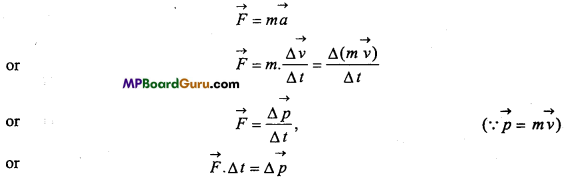or F.Δt = F.Δp
Hence, Impulse of the force = Change in momentum. Proved.

Question 8.
Prove that it is easier to pull a roller than to push.
Let force F is applied on a roller. Force F can be resolved into two components.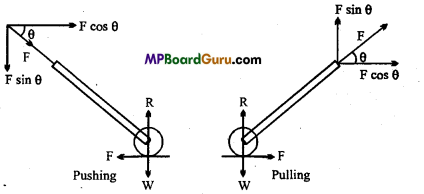• Horizontal component F cos θ and
• Vertical component Fsinθ.

At the time of pushing the roller, the components Fsin 8 increases the weight of the roller. While when roller is pulled the component Fsin 8 decreases the weight of the roller.

Question 9.
Find out recoiling velocity of gun.
Let the mass of the gun be Mand of the bullet is m moving with a velocity of ν1 and the recoil velocity of gun will be ν2 Before firing the momentum of gun and bullet will be zero and after firing the total momentum will be
2 + mν1
According to laws of conservation of linear momentum
Total linear momentum after firing = Total linear momentum before firing
2 + mν1 = 0
or Mν2 = 0 – mν1
or Recoil velocity of gun ν2 = – $$\frac{m v_{1}}{M}$$
Negative sign shows that gun moves backward when the bullet is fired.
Since m<<M
∴ ν2 < ν1 i-e-> Recoil velocity of gun is lesser than velocity of bullet.

Question 10.
State the principle of equilibrium of concurrent forces.
“If a number of forces act at the same point, they are called concurrent forces.”
Consider that a body is under the action of a number of forces. Suppose that the body is in equilibrium under the action of these forces, i.e., the body remains in its state of rest or of uniform motion along a straight line, when acted upon these forces.
“The condition that the body may be in equilibrium or the number of forces acting on the body may be in equilibrium is that these forces should produce zero resultant force.”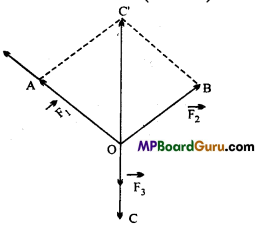Consider three concurrent forces $$\vec{F}_{1}, \vec{F}_{2}$$ and $$\vec{F}_{3}$$acting at the point ‘O’ (see Fig.).
In order to find the resultant of $$\vec{F}_{1}, \vec{F}_{2}$$ and $$\vec{F}_{3}$$, we first find the resultant of $$\vec{F}_{1}$$ and $$\vec{F}_{2}$$ by completing the parallelogram OAC’B. The diagonal OC’ of the parallelogram OAC ‘B gives
$$\vec{F}_{1}+\vec{F}_{2}$$ i.e., the resultant of $$\vec{F}_{1}$$ and $$\vec{F}_{2}$$ . If F$$\vec{F}_{3}$$ (represented by OC) as equal and opposite to
$$\vec{F}_{1}+\vec{F}_{2}$$ (represented by $$\overrightarrow{o \vec{C}})$$ then resultant of force $$\vec{F}_{1}$$, $$\vec{F}_{2}$$ and $$\vec{F}_{3}$$ acting at point O will be zero. Therefore, for $$\vec{F}_{1}$$,$$\vec{F}_{2}$$ and $$\vec{F}_{3}$$ to be in equilibrium, $$O \vec{C}=O \vec{C}^{\prime}$$
or $$\vec{F}_{3}=-\left(\vec{F}_{1}+\vec{F}_{2}\right)$$
or F$$\vec{F}_{1}$$+$$\vec{F}_{2}$$+$$\vec{F}_{3}$$ = 0
The condition for equilibrium of three concurrent forces is triangle law
of addition of vectors. Suppose that the forces $$\vec{F}_{1}$$,F$$\vec{F}_{2}$$ and $$\vec{F}_{3}$$ are such that they can be represented by the three sides $$\overrightarrow{A B}, \overrightarrow{B C}$$ and $$\overrightarrow{C A}$$ of the triangle ABC taken in same order, i.e., $$\overrightarrow{A B}=\vec{F}_{1}, \overrightarrow{B C}=\vec{F}_{2}$$ and $$\overrightarrow{C A}=\vec{F}_{3}$$ According to the triangle law of addition of vectors,
$$\overrightarrow{A B}+\overrightarrow{B C}=\overrightarrow{A C} \text { or } \overrightarrow{A B}+\overrightarrow{B C}-\overrightarrow{A C}$$ = 0
Now the vectors AC and CA are equal in magnitude but opposite in direction.
or $$\overrightarrow{A C}=-\overrightarrow{C A}$$
Hence, the eqn. (2) becomes .
$$\overrightarrow{A B}+\overrightarrow{B C}+\overrightarrow{C A}=0 \text { or } \vec{F}_{1}+\vec{F}_{2}+\vec{F}_{3}$$ = 0
Therefore, the resultant of three concurrent forces will be zero and hence they will be in equilibrium, if they can be represented completely by the three sides of a triangle taken in same order.
In general, the concurrent forces, $$\vec{F}_{1}, \vec{F}_{2}, \vec{F}_{3} \quad \ldots . . \vec{F}_{n}$$ Fn be in equilibrium, if their
resultant is zero, i.e.,
$$\vec{F}_{1}+\vec{F}_{2}+\vec{F}_{3}+\ldots .+\vec{F}_{n}$$ = 0 …(3)
In case, a number of forces act at a point, then they will be in equilibrium, if they can be represented completely by the sides of a closed polygon taken in order.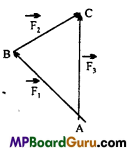Question 11.
State law of conservation of momentum and derive third law of motion with the help of this law.
Law of conservation of momentum : Law of conservation of momentum: In the absence of external force the linear momentum of a system remains always constant.
Proof: Let a force $$\vec{F}$$ is applied on a system for time interval dt, so that its momentum changes by dp.
∴ By Newton’s second law, F = $$\frac{d p}{d t}$$
If the system is in equilibrium, then the sum of all the forces acting on it will be zero,
F = 0
∴ $$\frac{d p}{d t}$$= 0
or p = constant, (by differential calculus, that $$\frac{d}{d t}$$ const. = 0)
Thus, in the absence of external force the linear momentum of a system is always conserved.

Let the initial momentum of two bodies A and B are $$\vec{p}_{1}$$ and $$\vec{p}_{2}$$, respectively interact.
Body B applies force on A is $$\overrightarrow{F_{12}}$$ and body A applies force $$\overrightarrow{F_{21}}$$ on body B.
Due to interaction the change in momentum are Δ $$\vec{p}_{1}$$ and Δ $$\vec{p}_{2}$$
∴ By the law of conservation of momentum,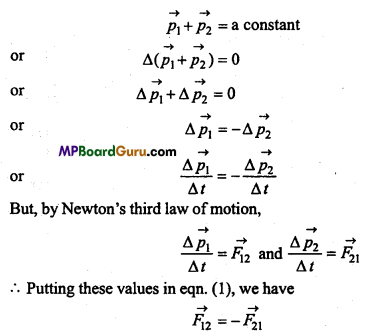Hence, force applied on A by B is equal and opposite to that of 5 by A. This is Newton’s third law of motion.

Question 12.
Write the laws of friction.
Limiting friction :
When a force is applied to a body to move on a surface or another body, the position at which the body is about to move on, is called the limiting equilibrium and the friction force acting is called limiting friction.

Laws of limiting friction :

• Friction force always opposes the motion of the body. Its direction is always opposite to the direction of motion.
• The limiting friction depends upon the nature of the two surfaces.
• The limiting friction does not depend upon the area of contact or shape of the two surfaces if normal reaction is constant.
• The magnitude of limiting friction fs is directly proportional to the normal reaction.

i.e., fs ∝ R
or fs = µsR
Where, µs is a constant called the coefficient of static friction.
µs = $$\frac{f_{s}}{R}$$

Question 13.
Write difference between Sliding friction and Rolling friction.
Difference between Sliding friction and Rolling friction:

 Sliding friction Rolling friction 1. When a body slides on another body, the friction force acting between the surfaces of contact is called sliding friction. 1. When a body rolls over the surface of another body, the friction force acting between the body and surface is called rolling friction. 2. The same surfaces are always in contact. 2. The different parts of the rolling body come into contact of the surface. 3. The sliding friction is greater than rolling friction. 3. The rolling friction is lesser than sliding friction.

Question 14.
Define angle of friction and angle of repose prove that they are equal to each other.
Angle of Friction : The angle between the resultant of limiting friction and the normal reaction is called angle of friction.
μs = tan λ …(1)

Angle of Repose : When a body is in limiting equilibrium on an inclined plane, then the inclination of the plane with the horizontal is called angle of repose.
μs = tan θ …(2)
From eqns. (1) and (2),
tan λ = tan θ
λ = θ
i.e., angle of friction is equal to angle of repose. Proved.

Question 15.
Explain the types of friction.
Frictions is of two types :
1. Static friction :
When a body tends to move on a surface but does not move, then the friction force acting between the surface is called static friction. The maximum value is called limiting friction.

2. Dynamic friction :
When a body moves on the surface, the friction force acting between the surface is called dynamic friction.
Dynamic friction is of two types :
(i) Sliding friction
(ii) Rolling friction.

(i) Sliding friction:
When a body slide over on another body, the friction force acting between the surfaces of contact is called sliding friction.
(ii) Rolling friction :
When a body roll over on another body, the friction force between the body and surface is called rolling friction.Question 16.
What is angle of friction? Prove that the tangent of angle of friction is equal to the coefficient of friction.
Angle of friction : The angle between the resultant of limiting friction and the normal reaction is called angle of friction.
Let a body is in the position of limiting friction on a surface.
Now, complete the parallelogram OACB.
∴ OC will represent the resultant of R and fs.
∴ Angle of friction ∠AOC = λ
Now, in right angle Δ OAC.
tan λ = $$\frac{A C}{O A}=\frac{O B}{O A}=\frac{f_{s}}{R}$$
But $$\frac{f_{s}}{R}$$ = μs
∴ μs = tan λ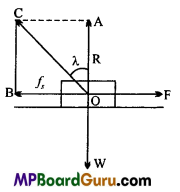Question 17.
What is angle of repose? Prove that the tangent of angle of repose is equal to coefficient of friction.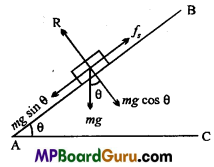Angle of Repose: When a body is in limiting equilibrium, on an inclined plane, then the inclination of the plane with the horizon is called angle of repose.
Let a body is in limiting equilibrium on an inclined R plane, its mass is m and angle of inclination is θ.
Now, the forces acting on the body are :

• mg acting vertically downward.
• Normal reaction R.
• Limiting forces fs, along the plane upward.

Now, resolving the weight mg, we get
R = mg cos θ and
fs = mg sin θ
Dividing eqn. (2) by eqn. (1), we get
$$\frac{f_{s}}{R}=\frac{m g \sin \theta}{m g \cos \theta}$$ = tan θ
But, $$\frac{f_{s}}{R}$$ = μs
μs = tan θ

Question 18.

• We can walk on earth with the help of friction. Good grip and working with the hand as holding pen, writing over paper, etc., are possible due to friction.
• Speed of the vehicle increases due to friction.
• The brakes work on the friction.
• Burning of matchsticks.
• Belts are used in the machines to transfer the force.
• Nails can be fixed on the wall or wood. Also, knots of ropes are due to friction force.

• The efficiency of the machines are reduced.
• Large amount of energy is wasted in the form of heat energy.
• It causes wear and tear in the machines.

Laws of Motion Class 11 Important Questions Long Answer Type

Question 1.
Explain laws of conservation of linear momentum. Prove that in absence of enternal forces, linear momentum of a moving particles is constant.
According to the laws of conservation of linear momentum, in an isolated system the vector sum of the linear momentum of all the bodies of the system is conserved and is not affected due to their mutual action and reaction.

(1) For a system of one particle : According to Newton’s second law,
Force acting upon a particle = Rate of change in momentum
i.e., $$\vec{F}=\frac{\Delta \vec{p}}{\Delta t}$$
If force acting upon the particle is zero i.e., $$\vec{F}$$ = 0,
Then, $$\frac{\Delta p}{\Delta t}$$ = 0
or
$$\Delta \vec{p}$$ = 0
or
$$\vec{p}$$ = Costant
i.e., in absence of external force, total momentum p is constant.

(2) For two particle system : Consider a two particle system in which a particle
execute F2 force on another particle and second particle execute F1 force on first particle. If change in momentum of both particles are ΔP1 and ΔP2, then according to Newton’s second
law,
Force = Rate of change in momentum
∴ Force on first particle $$\vec{F}_{1}=\frac{\Delta \overrightarrow{p_{1}}}{\Delta t}$$
and Force on second particle $$\vec{F}_{2}=\frac{\Delta p_{2}}{\Delta t}$$
But according to Newton’s third law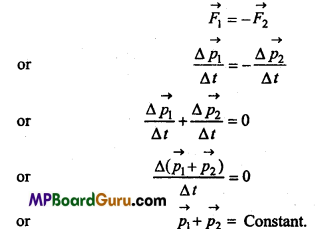Question 2.
Derive the expressions for the maximum speed in circular motion for safe
driving when
(i) Friction force Is zero and
Let a car of mass m is moving on a banked road in the circular path of radius r1 as shown in the figure. Then the forces acting on the car will be:

• Weight mg of car acting downwards.
• R, normal reaction at the angle θ with the vertical.
• $$\frac{m v^{2}}{r}$$, the centnpetal force radially inwards.
• ,F, the friction force between tyre and road.

Now, reaction R can be resolved into two components

• R cosθ along the vertical and
• R sinθ along the horizontal.

Also, F can be resolved into two components:

• F cosθ, along the horizontal and
• F sinθ, along the vertical.

Hence, we have
R cosθ = mg + F sinθ ….(1)
R sinθ + F cosθ = $$\frac{m v^{2}}{r}$$ ….(2)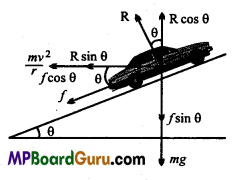and F= μR …(3)
Where, μ is coefficient of kinetic friction.
Now, from eqn. (1),
mg=R cosθ – F sinθ ….(4)
Dividing eqn. (2) by eqn. (4), we get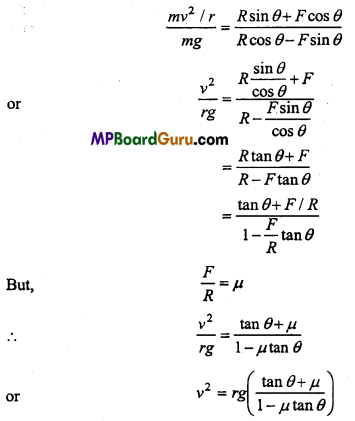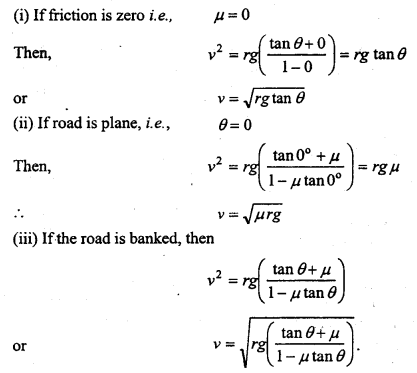Laws of Motion Class 11 Important Numerical Questions

Question 1.
Give the magnitude and direction of the net force acting on:
(a) A drop of rain falling down with a constant speed
(b) A cork of mass lOg floating on water
(c) A kite skillfully held stationary in the sky
(d) A car moving with a constant velocity of 30 km/h on a rough road
(e) A high speed electron in space far from all gravitating (material) objects and
free of electric and magnetic fields.
Solution:
(a) Net force acting on the drop of rain falling down with a constant speed will be zero. Weight of drop will be balanced by upthrust of air and viscous force.

(b) Net force acting on the cork will be zero, as its weight is balanced by the upthrust (i.e. weight of the water displaced).

(c) As the kite is held stationary, net force on the kite is zero. The force exerted on kite is balanced by its weight and tension in the string.

(d) Force is being applied to overcome the force of friction between the road and tyres. But as velocity of the car is constant, its acceleration a = 0 and hence net force = ma = 0.

(e) Net force on the high speed electron will be zero, since no gravitational/magnetic field is acting on it.

Question 2.
A constant retarding force of 50N is applied to a body of mass 20kg moving initially with a speed of 15ms-1. How long does it take to stop?
Solution:
Given :F = -50N (-ve sign shows that it is retarding force)
m = 20kg, u = 15m/s, ν = 0, t = ?
∴ F = ma
∴ -50 = 20 × a
-50 „ c . 2
⇒ a = $$\frac{-50}{20}$$ = -2.5m/s2
From ν = u +at
0 = 15-2.5t
∴ t = $$\frac{15}{2 \cdot 5}$$ = 6 sec.Question 3.
A body of mass 5.0kg is acted upon by two perpendicular forces 8N and 6N. Give the magnitude and direction of the acceleration of the body.
Solution: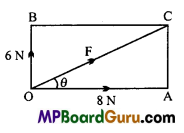Given : m 5kg, a = ?
Resultant of 8N and 6N will be
F = $$\sqrt{(8)^{2}+(6)^{2}} \mathrm{~N}$$
or F = $$\sqrt{64+36}$$ = $$\sqrt{100}$$N
or, F = 10N
If the resultant force makes angle 9 with 8N force, then
tanθ = $$\frac{6}{8}$$ = 0.75
∴ θ = tan-1 (0.75) = 37°
This is the direction of resultant force and hence the direction of acceleration of the
body.
Using F = ma
10 = 5 × a
⇒ a = $$\frac{10}{5}$$ = 2m/s 2

Question 4.
A force produces an acceleration of 10 ms 2 on a trolley of mass 5 kg. Calculate the force.
Solution:
Given : m = 5 kg, a = 10 ms2.
Now, F = ma
= 5 × 10= 50 N.

Question 5.
A shell of mass 0.02 kg is fired from a gun of mass 100 kg with a velocity of 80 ms-1. Calculate the recoil velocity of the gun.
Solution:
Given : M = 100kg, m = 0.02kg, ν = 80ms-1.
If the velocity of recoil is V, then
MV + mν = 0
or
V = $$-\frac{m v}{M}$$
∴ V = $$-\frac{0 \cdot 02 \times 80}{100}$$ = 0.016ms-1Question 6.
Two balls of masses 40 kg and 20 kg are moving with velocities 10 ms-1 and 50 ms-1 respectively towards each other. They collide and embedded. What will be the velocity of the system?
Solution:
Given : m1 = 40 kg,m2 – 20 kg, ν1 10 ms -1, ν2 = 50 ms-1
Let the velocity of the system after collision = ν
∴By the law of conservation of momentum,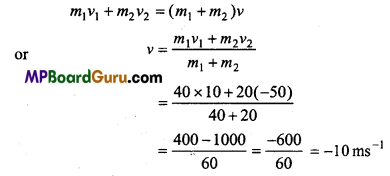Hence, 10ms-1 towards 20 kg mass.

Question 7.
A bullet of mass 10 gram moving with a velocity of 250m/s get embedded 2.5cm inside a wall. Find out the force exerted by the wall on bullet.
Solution:
Given : u = 250m/s, v =0,s = 2.5cm = 2.5 × 10-2 m,m = 10 gm= 0.01 kg From v2=u2+2as
0 = (250)2 + 2a × 2.5 × 10-2
or
a = $$\frac{-(250 \times 250)}{2 \cdot 5 \times 10^{-2} \times 2}$$
= -1.25 × 106m/s2
∴ Force exerted by wall on bullet
F = m.a = 0.01 × ( – 1.25 × 106) = -1.25 × 104 N.

Question 8.
A force of 10 N acts upon a body of mass 5 kg for 5 second Calculate :
(i) Impulse,
(ii) Change in momentum,
(iii) Change in velocity,
(iv) Acceleration.
Solution:
Given : m = 5 kg, F = 10 N, Δt = 5 sec
(i) Impulse = F.Δt
= 10 × 5 = 50 N-s.

(ii) Change in momentum = Impulse
= 50 kg m/s.

(iii) Change m velocity = $$\frac{\text { Change in momentum }}{\text { Mass of object }}$$
= $$\frac{50}{5}$$ = 10 m/s.

(iv) Acceleration a = $$\frac{F}{m}$$
or a = $$\frac{10}{5}$$ = 2m/s2

Question 9.
A driver can stop a car by applying a brake in 4 sec. If the car were running with a speed of 36 km/hr. Calculate the retarding force on the car, if the mass of the car is 400 kg and the mass of the driver is 65 kg.
Solution:
Given : ν (0) = 36 km/hr.
= $$\frac{36000 \mathrm{~m}}{60 \times 60 \mathrm{~s}}$$ = 10m/s
ν (t) = 0, t = 4 s, m = 400 + 65 = 465 kg.
Using formula ν (t) = ν (0) + at, we have
0= 10 + a × 4
or 4a = -10
∴ a = –$$\frac{10}{4}$$ -2.5m/s2
From formula F=ma
= 465 × (-2.5) = – 1162.5 N
∴ Retarding force = 1162.5 N.

Question 10.
In a rocket, the fuel is burning at the rate of 01 kg × s-1. The velocity of gas ejecting is 2 kms-1. Calculate the thrust on the rocket.
Solution:
Given:
ν = 2 km × s-1 = 2 × 103 ms-1.
∴ $$\frac{\Delta m}{\Delta t}$$ = 0.1 kg × s-1
Now, change in momentum per second = $$\frac{\Delta m}{\Delta t}$$× ν
= 0.1 × 2 × 103
=200kg ms-2
∴ Force on gas = Rate of change of momentum.
= 200 N
But, Thrust on rocket = Force on gas
= 200 N.

Question 11.
A body moving in uniform motion is stopped by applying force of 2000 N in $$\frac{1}{4}$$ seconds. Find out initial momentum of the body?
Solution:
Given : F = 2000 N, Δt = $$\frac{1}{4}$$ sec = 0.25 sec
Change in momentum Δp = F. Δt
Let p1 and p2 be initial and final momentum.
Then, Δp = p1 -p2 = p1 – 0 = p1
∴ P1 = F.Δt
= 2000 × $$\frac{1}{4}$$ = 500 N-s.Question 12.
Two circular bodies of mass 40 kg and 10 kg are moving with a velocity of 5 m/s and 20 m/s. Their direction is face to face. When they collide with each other forms a system. Find the velocity of the system.
Solution:
Given: m1=40 kg, m2= 10 kg, ν1= 5 m/s, ν2= -20 m/s, (since direction on is opposite) If ν is the velocity of the system, then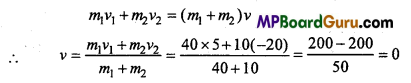or ν = 0
i.e., the system will get stoped.

Question 13.
A bomb of mass 25 kg moving with a velocity of 10 m/s get bombaded into two piece of masses 15 kg and 10 kg. If the velocity of big piece is zero then find the velocity of small piece.
Solution:
Given : M = 25 kg, ν = 10 m/s,
Initial momentum of the bomb = Mν = 25 × 10
= 250 kg m/s.
After bombarding m1 = 15 kg, m2= 10 kg, ν1= 0, ν2 = ?
Total momentum =15 × 0 + 10 × ν2
According to laws of conservation of momentum
10ν2 = 250
or
ν2 = $$\frac{250}{10}$$= 25m/s

Question 14.
A force of 72 N acting upon a body at an angle of 60° from the horizontal of mass 9 kg. Find out the acceleration of it in horizontal direction.
Solution:
Given :F= 72 N, θ=60°, m = 9 kg .
Component of force in horizontal direction FH=F cosθ
or FH = 72 × cos60° = 72 × $$\frac{1}{2}$$ = 36 N
∴ Acceleration in horizontal direction a = $$\frac{F_{\mathrm{H}}}{m}=\frac{36}{9}$$
= 4/ms2

Question 15.
A body of mass 0.25 kg moving with a velocity of 12 m/s is stopped by applying a force of 0.6 N. In how much time the body will stop and what will be the impulse?
Solution:
u = 12 m/s, m = 0.25kg, F = 0.6 N
Let the time be t in which the body get stopped.
From a= $$-\frac{F}{m}$$,weget
a = $$\frac{-0 \cdot 6}{0 \cdot 25}$$ = $$\frac{-12}{5}$$ m/s
Again from ν = u + at, we get
0 = 12 – $$\frac{12}{5}$$t
or $$\frac{12}{5}$$t = 12
or t = 5sec
and Impulse = F × Δt
0.6 × 5 =3N-s

Question 16.
A 10 gram bullet is shot from a 5 kg gun with a velocity of 400 m/s. What is the speed of recoil of the gun ?
Solution:
Given : m1 = 10g = 10-2kg, m2= 5kg, ν1 400m/s, ν2 = ?
From m1ν1= m2ν2, we get
ν2 = $$-\frac{m_{1} v_{1}}{m_{2}}$$
or ν2 = $$\frac{-10^{-2} \times 400}{5}$$
or ν2 = -0.8 m/s .

Question 17.
A man of mass 70 kg stands on a weighing machine in a lift, which is moving:
(a) Upwards with a uniform speed of 10m/s.
(b) Downwards with a uniform acceleration of 5m/s2.
(c) Upwards with a uniform acceleration of 5m/s2.
What would be the readings on the scale in each case?
(d) What would be the reading if the lift mechanism failed and it hurtled down freely under gravity
Solution:
Given : m = 70kg; g = 10m/s2
(a) Lift is moving upwards with uniform speed, its acceleration = 0
∴ R = mg = 70 × 10 = 700N.
∴ Reading of the scale = $$\frac{700}{10^{\circ}}$$ = 70 kg

(b) Lift is moving downwards with uniform acceleration a = 5m/s2
∴ mg – R = F
⇒ ma = mg – R
⇒ R=m(g – a) = 70 × (10 – 5)
R = 350N.
∴ Reading of the scale = $$\frac{350}{10}$$ = 35

(c) Lift is moving upwards with uniform acceleration a = 5m/s2, then
R – mg = F
⇒ R = ma + mg, [∴F = ma]
⇒ R = m(g + a) = 70 × (10 + 5)
or R = 1050N
∴ Reading of the scale = $$\frac{1050}{10}$$
= 105kg

(d) When the lift falls freely under gravity, then a = g
Using, R = m(g – a)
R = 70(g – g) = 0.
Thus the reading on the scale is zero, this is the state of weightlessness.Question 18.
Two masses 8kg and 12kg are connected at the two ends of a light inextensible string that passes over a frictionless pulley as shown in figure. Find the acceleration of the masses and the tension in the string, when the masses are released.
Solution:
Let a be the acceleration of the bodies and T be the tension in the string. Then, equation of motion for mass m1
T – m1g = m1a …(1)
For mass m2
m2g – T = m2a …(2)
On adding eqns.(1)and(2), and putting the value of m1 =8 kg,m2= 12kg and g 10ms-2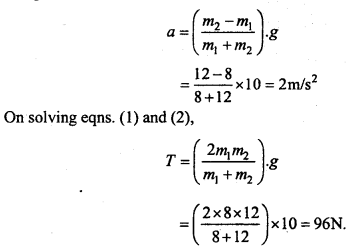Question 19.
When a force of 50 kg weight applied on a sledge of mass 500 kg then it tends to move. Find out the coefficient of friction between it.
Solution:
Given, fs = F =50 kg – wt =50 × g – N, R = 500g – N
From μs = $$\frac{f_{s}}{\mathrm{R}}$$ we get
μs = $$\frac{50 \mathrm{~g}-\mathrm{N}}{500 \mathrm{~g}-\mathrm{N}}$$ = 0.1

Question 20.
A mass of 2 kg is placed on a rough inclined plane of angle 30°. If coefficient of friction is 0.7 then find out the resultant friction force, acting on the block.
Solution:
Given, m = 2 kg, θ = 30°, μs = 0.7
From μs = $$\frac{f_{s}}{\mathrm{R}}$$ we get
fs = μs.R
or
fs = μs.mg cosθ
or fs = 0.7 × 2 × 9.8 ×cos30°
= 0.7 × 2 × 9.8 ×$$\frac{\sqrt{3}}{2}$$
= 6.86 × 1.732
= 11.88N

Question 21.
A block of 1 kg is resting on the plane of a truck. If the coefficient of friction between them is 0-6 and the acceleration of the truck is 10m/s2. Find out the friction force acting on the block.
Solution:
Given : = μs = 0.6,a = 10m/s2,m1 = 1kg
∴ Frictional force acting upon the block = μs.m1g – m1a
= 0.6 × 1 × 9.8-1 × 10
= 5.88 – 10
= -4.12 N.

Question 22.
A man of mass 100 kg is slipping from an electric pole. If the frictional force is 480 N, then find out the acceleration of the mass. Given g = 9.8 m/s2
Solution:
Given, m = 100kg, frictional force F = 480 N, g = 9-8 m/s2 Let the acceleration of the mass be a.
∴ From second laws of motion
ma = mg – F
or a = g – $$\frac{F}{m}$$
or a = 9.8 – $$\frac{480}{100}$$
or a = 9.8 – 4.8
or a =5 m/s2.

Question 23.
A horizontal force of 1.2 kg-wt. is applied on a 3 kg block, which rest on a horizontal surface. If the coefficient of friction is 0.2, find the acceleration produced in the block.
Solution:
Given, m – 3 kg, μ = 0.2,F = 1.2 kg-wt. = 1.2 × 9.8 N.
Let the acceleration of the block be a.
μ = $$\frac{f_{k}}{m g}$$ we get
fk = μ.mg = 0.2 × 3 × 9.8 = 5.88 N
Again from f= F – fk, we get
f =1.2 × 9.8 – 5.88 N
or f =11.76 – 5.88 = 5.88 N
∴ Acceleration a = $$\frac{f}{m}=\frac{5 \cdot 88}{3}$$ = 1.96m/s2Question 24.
A car is moving with a velocity of 20 m/s on a plane road. If the coefficient of friction between tyre and road is 0.4. Find out the distance after which the car will get stopped, (g = 10 m/s2)
Solution:
Given, u = 20 m/s, μ = 0.4,g = 10 m/s2
Let the retardation of the car be a and its mass be m.
From μ = $$\frac{\mathrm{F}}{R}$$ we get
μ = $$\frac{m \cdot a}{m \cdot g}=\frac{a}{g}$$
or a = μ.g = 0.4 × 10 = 4m/s2
Let the car get stopped after travelling s distance.
∴ From v2 = u2 – 2as, we get
0 = u2 – 2as
or s = $$\frac{u^{2}}{2 a}=\frac{20^{2}}{2 \times 4}$$
= 50m

Question 25.
A body of mass 4 kg ¡s tied with a string and rotated in a circular path of diameter 8 m. If the body Is moving with uniform peed of 600 m per minute, calculate the centripetal acceleration and centripetal force.
Solution:
Given:m = 4kg,r=$$\frac{8}{2}$$ = 4m,ν = 600m × min-1
= $$\frac{600}{60}$$ ms -1
= 10 ms -1
∴ Centripetal acceleration = $$\frac{v^{2}}{r}=\frac{10 \times 10}{4}$$ =25ms-1
and Centripetal force = $$\frac{m v^{2}}{r}$$ = 4 × 25 = 100 N

Question 26.
The mass of scooter and driver moving with a speed of 36 km x h-1 is 150 kg, suddenly the scooter takes a turn at the radius of 30 m. What will be the horizontal force to make it possible?
Solution:
Given : r=30m,m = 150 kg and ν = 36km x h-1
ν = $$\frac{36 \times 1000}{60 \times 60}$$ = 10ms-1
∴ Horizontal force = $$\frac{m v^{2}}{r}$$
= $$\frac{150 \times 10^{2}}{30}=\frac{150 \times 100}{30}$$ = 500 N

Question 27.
A car of mass 1600 kg moving In a circular horizontal road of radius 20 m, with a speed 12.5 ms-1. What should be the friction force between car and road, so that the car does not skid?
Solution:
Given: m = 1600 kg ,r =20 m , ν = 12.5 ms-1.
Now, friction force, F = $$ms-1$$
∴  F = $$\frac{1600 \times(12 \cdot 5)^{2}}{20}$$ = 1.25 x 10N
Again F = µrg
or µ = $$\frac{F}{r g}$$
= $$\frac{1 \cdot 25 \times 10^{4}}{20 \times 9 \cdot 8}$$ = 63.78

Question 28.
A boy ties a stone of mass 0.5 kg at one end of 40 cm string and whirled it in a vertical circle. The speed of the stone at the lowest point of the circle is 3m/s. Find the tension at this point.
Solution:
Given : mass m = 0.5 kg, length of string r = 40 cm = 0.4m, velocity at lowest point VL= 3m/s.
Tension at lowermost point TL = mg + $$\frac{m V_{L}^{2}}{r}$$
= 0.5 × 10 + $$\frac{0 \cdot 5 \times 3 \times 3}{0 \cdot 4}$$
= 5 + $$\frac{45}{4}$$ = 5 + 11.25 = 16.25N.

Question 29.
What will be the maximum speed of a car in a circular path of radius 30 m, if the coefficient of friction between tyre and road is 0.4
Solution:
Given : r = 30 μ= 0.4
Now, ν = $$\sqrt{\mu r g}$$
= $$\sqrt{0 \cdot 4 \times 30 \times 9 \cdot 8}$$= 10.84 ms-1.

Question 30.
A particle completes 7 revolutions in 100 seconds while moving with a uniform speed in a circular groove of 12 cm radius. Calculate its angular speed, linear speed and centripetal acceleration.
Solution:
7 revolutions are completed in 100 second.
1 revolution is completed in time-period T = $$\frac{100}{7}$$ sec:
Angular speed ω = $$\frac{2 \pi}{T}=\frac{2 \times \pi \times 7}{100}=\frac{2 \times 22 \times 7}{10 \times 70}=\frac{44}{100}$$
Linear speed v = = ωr = 0.44 × 12 = 5.28 cm/sec.
Centripetal acceleration a= (ω2r = $$\frac{44}{100}$$ ×$$\frac{44}{100}$$ × 12 = 2.32 cm/s2.Question 31.
A string of 1 m can resist a force of 100 N. One end of this string is tied with a stone of mass 1 kg and whirled in horizontal plane. Calculate the maximum linear speed, so that string does not break.
Solution:
Given : centripetal force = 100 N, m = 1 kg and r = 1 m.
Now, centripetal force, F = $$\frac{m v^{2}}{r}$$
or 100 =$$\frac{1 \times v^{2}}{1}$$
or ν2 = 100
∴ ν = $$\sqrt{100}$$ = 10ms-1

Laws of Motion Class 11 Important Questions Objective Type

1. Multiple- choice questions:

Question 1.
The first law of motion is also known as :
(a) Momentum
(b) Laws of inertia
(c) Action-reaction
(d) None of these.
(b) Laws of inertia

Question 2.
The measure of inertia is its :
(a) Mass
(b) Velocity
(c) Both
(d) None of these.
(a) Mass

Question 3.
If net force in a body is zero, then its acceleration :
(a) Will increase
(b) Will decrease
(c) Will be zero
(d) None of these.
(c) Will be zero

Question 4.
One Newton is equal to :
(a) kg × metre × time
(b) kg × $$\frac{\text { metre }}{\text { second }}$$
(c) metre × second
(d) kg × second
(b) kg × $$\frac{\text { metre }}{\text { second }}$$

Question 5.
If constant force is acting upon a body, then which will be among uniform :
(a) Velocity
(b) Acceleration
(e) Momentum
(d) Kinetic energy.
(b) Acceleration

Question 6.
Rocket propulsion is based on :
(a) First laws of motion
(b) Laws of conservation of momentum
(c) Second laws of motion
(d) None of these.
(b) Laws of conservation of momentum

Question 7.
Limiting friction depends upon :
(a) Area of the surface
(b) Size of the surface
(c) Nature of surface
(d) Normal Reaction.
(c) Nature of surfaceQuestion 8.
Weight of a person in a lift will be maximum, when lift moves :
(a) Upward
(b) Downward
(c) Independently
(d) None of these.
(a) Upward

Question 9.
From which laws of Newton’s, the other two laws can be deduced :
(a) Newton’s first law
(b) Newton’s second laws
(c) Newton’s third law
(d) None of these.
(b) Newton’s second laws

Question 10.
If a lift is falling independently, the weight of the person of 100 kg will be:
(a) 880 joule
(b) 980 Newton
(c) 980 kg
(d) Zero.
(d) Zero.

Question 11.
Formula for coefficient of static friction is: .
(a) fs × R
(b) R | fs
(c) μs = fs ×R
(d) μs = fs
(c) μs = fs ×R

Question 12.
Relation between angle of friction and angle of repose is :
(a) tanθ + tanλ
(b) tanθ = tanλ
(c) tanθ × tanλ
(d) tanθ ÷ tanλ
(b) tanθ = tanλ

Question 13.
On applying a force on a trolley of 5 kg, acceleration produced is 10 m/s2, then the applied force will be :
(a) 100 Newton
(b) 50 Newton
(c) 25 Newton
(d) 20 Newton.
(b) 50 Newton

Question 14.
A body of 1 kilogram falls freely on the surface of the ground, on touching ground the force applied by it is :
(a) 1 kilogram
(b) 1 kilogram-weight
(c) 2 kilogram
(d) 2 kilogram-weight.
(b) 1 kilogram-weight

Question 15.
When a force of 10 Newton acts upon a body of mass 5 kg for 5 second, then its impulse will be:
(a) 25 Newton × second
(b) 20 Newton × second
(c) 15 Newton × second
(d) 50 Newton × second.
(d) 50 Newton × second.2. Fill in the blanks:

1. One newton = ………………… dyne.
105

2. Force = Mass × ………………..
Acceleration

3. First laws of motion is called as ………………
Inertia

4. Rate of change in momentum is directly proportional to ………………….
Force

5. For every action there is equal and opposite …………………
Reaction

6. SI unit of force is …………………
Newton

7. Rocket propulsion is based on …………………. principle.
Conservation of momentum

8. C.G.S. unit of force is ………………….
Dyne9. 1 kilogram-weight = ………………….. Newton.
9.8

10. All the frames of reference in which Newtons laws hold true are called …………………….
Inertial frames

11. The force which does not act on the body but due to acceleration of frame of reference, appears to act on body is called ………………..
Fictitious force

12. Kinetic friction is always less than ………………….
Static limiting friction

13. The ratio of limiting static friction and normal reaction is called ………………….
Coefficient of limiting friction

14. Frictional force is independent of ………………..
Area of contact

15. Newton’s laws of motion doesn’t apply for …………………. frame.
Non-inertial

3. Match the following:
I

 Column ‘A’ Column ‘B’ 1. Change in momentum (a) Congruent force 2. Action and reactions is in (b) Impulse 3. Frictional force (c) Ball bearing 4. Forces whose line of action passes through same point (d) Force opposing motion 5. Reduces friction (e) Third laws of motion.

1. (b) Impulse
2. (e) Third laws of motion.
3. (d) Force opposing motion
4. (a) Congruent force
5. (c) Ball bearingII

 Column ‘A’ Column ‘B’ 1. Pasenger bent forward when suddenly bus stops (a) To reduce impulse 2. Detechment of particle from cloth on striking by rod (b) Action-reaction 3. Rocket propulsion . (c) Inertia of motion 4. Player’s take’s their hand backside while catching cricket ball (c) Inertia of motion 5. Waking in ground surface (d) Conservation of momentum

1. (c) Inertia of motion
2. (e) Inertia at rest.
3. (d) Conservation of momentum
4. (a) To reduce impulse
5. (b) Action-reaction

4. Write true or false:

1. Newton’s first law is laws of inertia.
True

2. Change in momentum is not directly proportional to applied force.
False

3. For every action there is equal and opposite reaction.
True

4. When a bullet penetrate inside a wood, its velocity remain conserved.
False

5. When the lift moves upward, weight of the person inside it, get decreased.
False

6. If the body is not in rest the net force acting upon it cannot be zero.
False

7. Bus moving in circular path is the example of non-inertial frame of reference.
True8. The body remains at rest when an external force acts upon it.
True

9. Walking in sand is difficult.
True

10. Weight of free falling body is not zero.
False

11. Momentum will be zero when brakes is applied on vehicle in motion.
True

12. Force is neccesary to maintain the body in motion with constant velocity.
False

13. It is easior to walk in smooth surface.
False

14. When a cork float on the surface of water, the net force acting is zero.
True

15. The earth extract gravitational force on moon.
True

16. Momentum remain conserved for a body thrown upward.
False1. Many forces act on a body can it stay in the state of rest.
Yes, if the resultant of all forces become zero.

2. Is the net force on a cork floating on water surface zero.